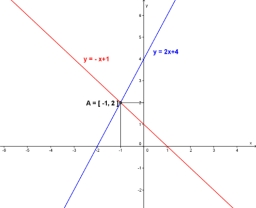# What is 19

What is the equation of the line whose x-intercept is - 3 and y-intercept are -4? Find coefficients A, B, C in normal line equation: Ax + By = C

A =  4
B =  3
C =  -12

### Step-by-step explanation:Did you find an error or inaccuracy? Feel free to write us. Thank you!

Showing 1 comment:
Iknownobody
Hello, sir/ma'am! Good day. Since intercepts have been given, I used intercepts form which is x/a +y/b=1, doing operation eventually I got -1/3 x -1/4 y =12 so A=-⅓ B=-¼ and C= 12...

Tips for related online calculators
Line slope calculator is helpful for basic calculations in analytic geometry. The coordinates of two points in the plane calculate slope, normal and parametric line equation(s), slope, directional angle, direction vector, the length of the segment, intersections of the coordinate axes, etc.
Do you have a system of equations and looking for calculator system of linear equations?